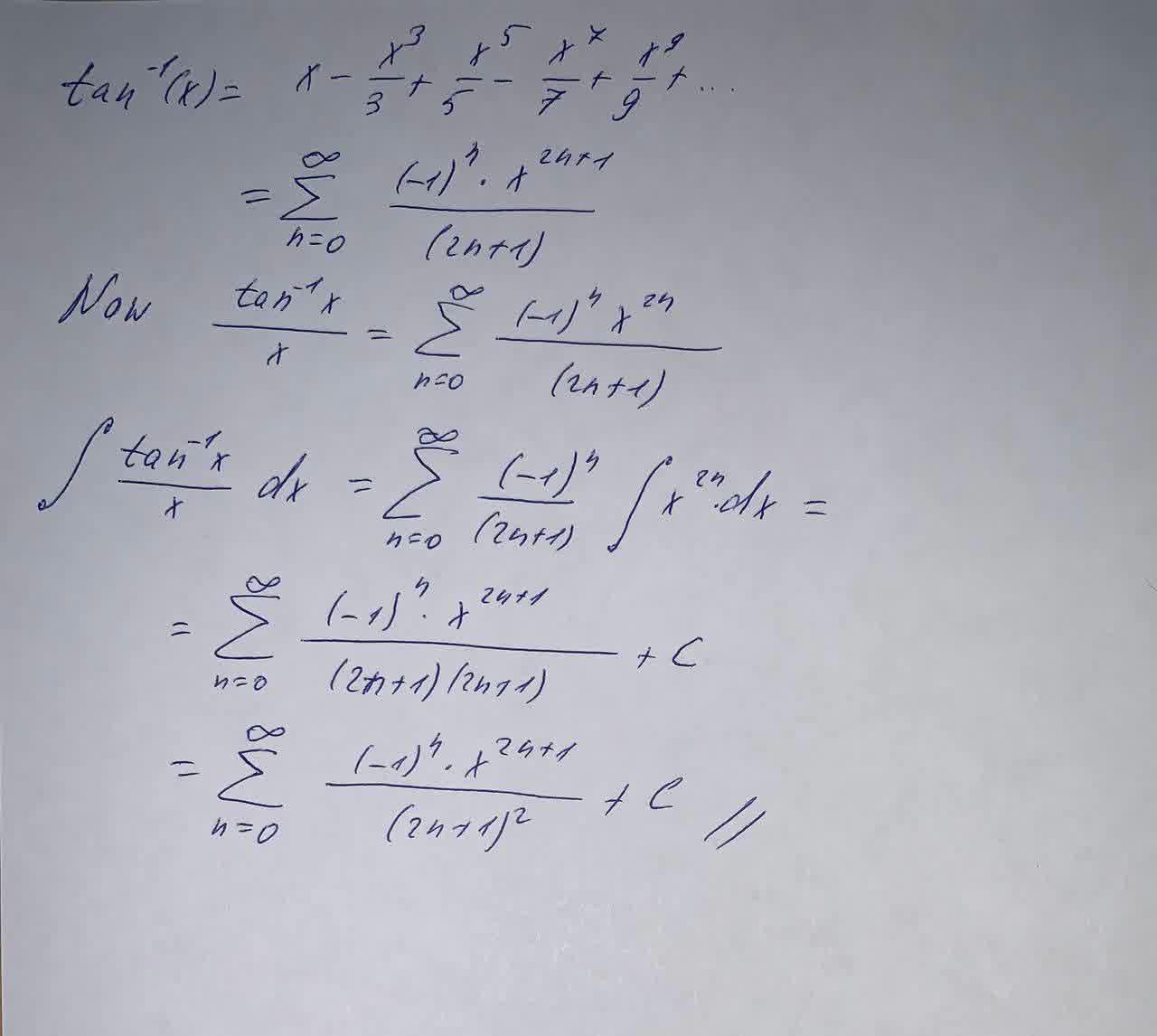# Evaluate the indefinite integral as a power series. \int \frac{\tan^{-1}x}{x}dx f(x)=C+\sum_{n=0}^\infty\left( \dots \right) What is the radius of convergence R?Dillard 2021-05-27 Answered
Evaluate the indefinite integral as a power series.
$\int \frac{{\mathrm{tan}}^{-1}x}{x}dx$
$f\left(x\right)=C+\sum _{n=0}^{\mathrm{\infty }}\left(\dots \right)$
What is the radius of convergence R?
You can still ask an expert for help

• Questions are typically answered in as fast as 30 minutes

Solve your problem for the price of one coffee

• Math expert for every subject
• Pay only if we can solve itpierretteA